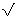#The Square Root of 2

Math Lair Home > Topics > The Square Root of 2

The square root of 2 to 100 decimals is: 1.4142135623730950488016887242096980785696718753769480731766797379907324784621070388503875343276415727.

The square root of 2 is the solution to the equation x² = 2, which results when using the Pythagorean Theorem to find the length of the hypotenuse of a right triangle where both of the legs are of length 1. Around 1700 B.C., the Babylonians found the approximation 1; 24; 51; 10 in their base 60 notation for the square root of 2, which works out to 1.414212963 in decimal notation. However, they likely didn't realise that2 was a different kind of number—an irrational number.

It was Pythagoras or the Pythagoreans who first were able to prove that this number was irrational. The proof follows:

Start by assuming the reverse of what we want to prove; that is, assume that2 is rational. Therefore, it must be equal to p/q, where p and q are some integers in lowest terms. If they weren't in lowest terms, we could cancel out any common factors and would then be able to write it in lowest terms. Now, square both sides, and we get:
2 = p²/q²
or 2q² = p²
Since the left-hand side is an even number, then the right-hand side must also be even. Therefore, p is even as well. We can write p = 2r, where r is some other integer. Then, we get
p² = (2r)² = 4r² = 2q²
So, q² = 2s².
Therefore, q² is also an even number, and so is q. But if p and q are both even, then they have not been reduced to their lowest common factor, contradicting one of our assumptions. Therefore, our initial assumption must be wrong, and the square root of 2 cannot be written as p/q, where p and q are integers. Therefore, the square root of 2 is irrational.

Here's another nice variation of that proof: As above, suppose that p² = 2q², where p and q are integers. Since each integer can be factored into primes, we could do that for p and q. Therefore, in p², there are a certain number of primes doubled up. And in q², there are also a certain number of doubled-up primes. But, in 2 x q² there is a 2 that has no partner. This is a contradiction.

Not too long after, Theatetus (417 B.C.–369 B.C.) proved that the square roots of 3, 5, and in general the square roots of any integer that isn't a square number are irrational.